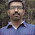I request GUEST WRITERS for this web site. Anyone interested please contact me at saravu2k2013@gmail.com Thanks

Set of solved exercises in Normalization / Normalization Solved Examples / How to find candidate keys, and primary keys in database? / Sets of examples to find the keys of a tables / Process of Key finding in a database - Examples

Question:

Consider a relation R(A, B, C, D, E) with FD's AB C, CD E, C A, C D, D B.
The possible candidate keys for R are AB, AD, C
(a) List all the functional dependencies that violate 3NF. If any, then decompose R accordingly.
(b) List all the FD's that violate BCNF. If any, then decompose R accordingly.

Solution (a):

To be in 3NF, the following points should not be violated by any of the FDs;

(i) R should be in 2NF;
According to the properties that are to be held for 2NF (No partial functional dependencies), all the non-prime attributes (E is the only non-prime attribute in our case) depend on prime attributes (A, B, C, and D in our case) or keys as a whole. No partial dependencies found. Hence, we would say that R is in 2NF.

(ii) Transitive functional dependencies of non-prime attributes on candidate keys are prohibited;
In the list of functional dependencies, we found no non-key dependencies. That is, no non-prime attribute is functionally dependent on another non-prime attribute.
From points (i) and (ii), it is clear that there are no FDs that violate 3NF. Hence, we would say that R is in 3NF.

Solution (b):

R can be in BCNF if and only if R is in 3NF and every determinant is a candidate key.

From the solution (a), you would understand that R is in 3NF. But every determinant is not a candidate key. In the given set of FDs, D B is the only functional dependency that violates this rule. That is, D is not a candidate key on its own. Hence, the relation R is not in BCNF.

To convert R into a BCNF relation, we need to decompose R using the FD D B. Then we shall get the following relations R1 and R2;

R1 (D, B) and R2 (A, C, D, E)

1.R2 must be R2(A,B,C,D,E) since the FD AB->C is lost

2.I have explained this exercise as an example for BCNF decomposition. A decomposition can be either dependency preserving or not. My example is not a dependency preserving decomposition. Thanks for your comment.

3.CD is also not a candidate key on its own. Then why not decompose on the basis of that?

1.It is given that C is one candidate key. While C is a candidate key then CD is a super key. Hence, the left hand side is a determinant. But in D --> B, D is not a candidate key.

SQL exercises for beginners one

SQL Exercises for Beginners / Simple SQL Exercises with Answers / Solved SQL exercises / SQL solved exercises with simple conditions / So...